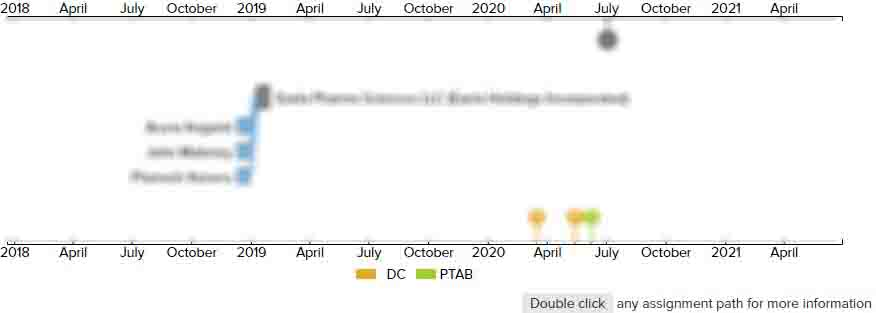# Adaptive block size image compression method and system

• US 5,452,104 A
• Filed: 08/04/1993
• Issued: 09/19/1995
• Est. Priority Date: 02/27/1990
• Status: Expired due to Term
##### First Claim
Patent Images

1. In an adaptive block size image compression system for compressing a block of input pixel data for transmission by performing a discrete cosine transform (DCT) operation on the block of pixel data and on at least one predetermined level of constituent sub-blocks thereof so as to produce corresponding image block and sub-blocks of DC and AC DCT coefficient values;

• determining for each image block and each corresponding group of image sub-blocks of DCT coefficient values a bit count corresponding to the number of bits required to respectively encode the image block and each corresponding group of image sub-blocks of DCT coefficient values according to a predetermined coding format;

determining from the bit count ones of the image block and corresponding group of image sub-blocks of DCT coefficient values requiring a lesser number of bits to encode according to the coding format;

selecting ones of the image block and image sub-blocks of DCT coefficients values requiring a lesser number of bits to encode according to the coding format so as to form a composite image block;

ordering the DCT coefficient values of the composite image block according to a predetermined ordering format;

encoding the ordered DCT coefficient values of the composite image block according to the encoding format; and

assembling the encoded ordered DCT coefficient values of the composite image block along with information identifying the selected ones of the image block and image sub-blocks of DCT coefficient values of the composite image block, a subsystem for providing further compression of the block of pixel data comprising;

discrete quadtree transform means for, receiving said input block of pixel data, performing a first discrete cosine transform (DCT) operation on said block of pixel data so as to produce a corresponding first block of DC and AC DCT coefficient values, performing a series of additional DCT operations with a first additional DCT operation performed on said DC DCT coefficients of said first block of DC and AC DCT coefficient values with each subsequent additional DCT operation performed on resultant DC coefficients values of a proceeding additional DCT operation with a last additional DC operation producing a corresponding last block of DC and AC DCT coefficient values, and providing an output of said last block of DC and AC DCT coefficient values;

code length determination means for, receiving each of said last block of DC and AC DCT coefficient values, generating a corresponding code length value corresponding to a length of each of said last block DC and AC DCT coefficient value as encoded according to said predetermined coding format, inserting each code length value in place of a DC DCT coefficient for each corresponding image block and image sub-block in said bit count determination, wherein said ones of said image block and image sub-blocks are selected for said composite image block using said corresponding code length values; and

replacement means for receiving each of said last block of DC and AC DCT coefficient values and replacing each DC DCT coefficient of said selected ones of said image block and image sub-blocks of said composite image block with a corresponding one of said last block DC and AC DCT coefficient values.

• ##### 0 Assignments
Timeline View
Assignment View×
×